# Advanced Geometry : How to find the area of a kite

## Example Questions

2 Next →

### Example Question #1 : How To Find The Area Of A Kite

Find the area of a kite with the diagonal lengths of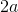and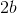.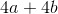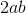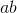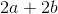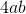Explanation:

Write the formula to find the area of a kite.  Substitute the diagonals and solve.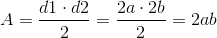### Example Question #2 : How To Find The Area Of A Kite

Find the area of a kite with diagonal lengths of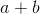and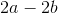.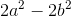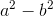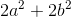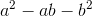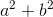Explanation:

Write the formula for the area of a kite.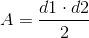Plug in the given diagonals.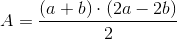Pull out a common factor of two inand simplify.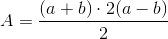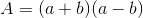Use the FOIL method to simplify.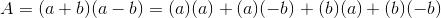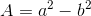### Example Question #41 : Kites

Find the area of a kite, if the diagonals of the kite are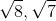.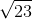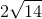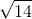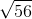Explanation:

You find the area of a kite by using the lengths of the diagonals.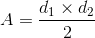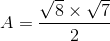, which is equal to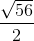You can reduce this to,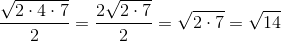for the final answer.

### Example Question #42 : Kites

Show algebraically how the formula of the area of a kite is developed.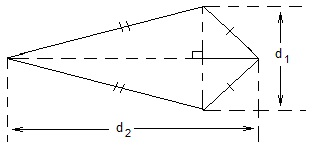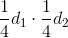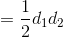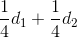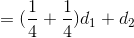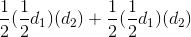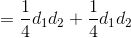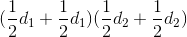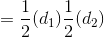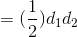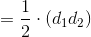Explanation:

1) The given kite is divded into two congruent triangles.

2) Each triangle has a height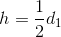and a base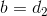.

3) The areaof each triangle is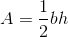.

4) The areas of the two triangles are added together,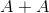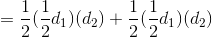### Example Question #41 : Plane Geometry

The rectangle area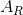is 220.  What is the area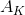of the inscribed

kite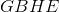?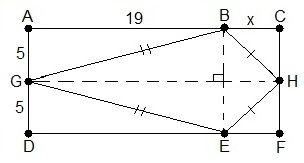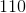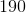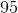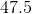Explanation:

1) The measures of the kite diagonals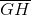and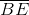have to be found.

2) Using the circumscribed rectangle,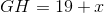, and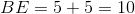.

3)has to be found to find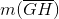.

4) The rectangle area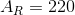.

5)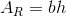.

6) From step 1) and step 2), using substitution,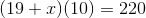.

7) Solving the equation for x,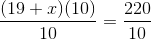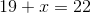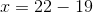8)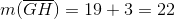9) Kite area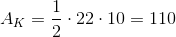2 Next →